The Cost of Trade Credit is an important interest rate that is calculated in the context of accounts payable management. This is because payables are a sources of working capital to the firm. It is important to manage this source of funding well and to be able to calculate the effective cost of trade credit.

To see why payables management is important, consider a situation where a firm pays its invoices before they become due. In that case, cash is used unnecessarily and interest is foregone. Similarly, if a company pays its bills late, this may damage relationships and lead to more restrictive credit terms in the future. Worse still, it could be that the company has to pay interest charges that are very high compared to other sources of short-term financing.

## Payables terms

Very often, invoices contain a discount if the company pays the bill quickly. One commonly used term is “2/10 net 60”. This means that if the bill is paid within 10 days, the company gets a 2% discount. If the company does not take advantage of the discount, it is expected to pay back the full amount 60 days from now. Another popular payables term is 2/10 net 30, in which case payment is due within 30 days, rather than 60.

## Cost of trade credit formula

To analyse whether it makes sense for a company to take advantage of the discount, we should calculate the cost of trade credit. Using the following formula, we can calculate the nominal annual cost of trade credit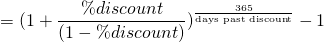where days past discount is the number of days after the end of the discount period.

## Cost of trade credit example

In general, when the cost is higher than other forms of credit, the company should pay the invoice on the last day of the discount period. To see this, let’s consider a simple example. Suppose we have an invoice with “2/10 net 60” terms. Let’s calculate the cost when we pay the bill at different points in time: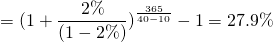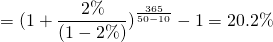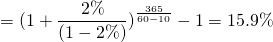As we mentioned earlier, it is best to pay the invoice on the last day of the discount period. If the invoice has not been paid by then, the company should wait until the last day of the due date. This is because the cost of credit is decreases as the payment period increases. The following figure reports the cost for different payment days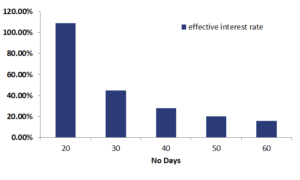## Summary

We calculate the implicit cost of trade credit which we can calculate using the payables terms. The cost of credit can easily be calculated using an Excel spreadsheet.